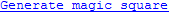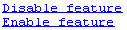## Create Hyperlinks that Run Functions

The special keyword `matlab:` lets you embed commands in other functions. Most commonly, the functions that contain it display hyperlinks, which execute the commands when you click the hyperlink text. Functions that support `matlab:` syntax include `disp`, `error`, `fprintf`, `help`, and `warning`.

Use `matlab:` syntax to create a hyperlink in the Command Window that runs one or more functions. For example, you can use `disp` to display the word Hypotenuse as an executable hyperlink as follows:

`disp('<a href="matlab:a=3; b=4;c=hypot(a,b)">Hypotenuse</a>')`
Clicking the hyperlink executes the three commands following `matlab:`, resulting in
```c = 5```
Executing the link creates or redefines the variables `a`, `b`, and `c` in the base workspace.

The argument to `disp` is an `<a href>` HTML hyperlink. Include the full hypertext text, from `'<a href=` to `</a>'` within a single line, that is, do not continue long text on a new line. No spaces are allowed after the opening `<` and before the closing `>`. A single space is required between `a` and `href`.

You cannot directly execute `matlab:` syntax. That is, if you type

`matlab:a=3; b=4;c=hypot(a,b)`
you receive an error, because MATLAB® interprets the colon as an array operator in an illegal context:
```??? matlab:a=3; b=4;c=hypot(a,b) | Error: The expression to the left of the equals sign is not a valid target for an assignment.```

You do not need to use `matlab:` to display a live hyperlink to the Web. For example, if you want to link to an external Web page, you can use `disp`, as follows:

`disp('<a href="http://en.wikipedia.org/wiki/Hypotenuse">Hypotenuse</a>')`
The result in the Command Window looks the same as the previous example, but instead opens a page at en.wikipedia.org:
`Hypotenuse`

Using `matlab:`, you can:

### Run a Single Function

Use `matlab:` to run a specified statement when you click a hyperlink in the Command Window. For example, run this command:

```disp('<a href="matlab:magic(4)">Generate magic square</a>') ```

It displays this link in the Command Window:When you click the link, MATLAB runs `magic(4)`.

### Run Multiple Functions

You can run multiple functions with a single link. For example, run this command:

`disp('<a href="matlab: x=0:1:8;y=sin(x);plot(x,y)">Plot x,y</a>')`

It displays this link in the Command Window:When you click the link, MATLAB runs this code:

```x = 0:1:8; y = sin(x); plot(x,y) ```

Redefine `x` in the base workspace:

`x = -2*pi:pi/16:2*pi;`

Click the hyperlink, `Plot x,y` again and it changes the current value of `x` back to `0:1:8`. The code that `matlab:` runs when you click the `Plot x,y` defines `x` in the base workspace.

### Provide Command Options

Use multiple `matlab:` statements in a file to present options, such as

```disp('<a href = "matlab:state = 0">Disable feature</a>') disp('<a href = "matlab:state = 1">Enable feature</a>') ```

The Command Window displays the links that follow. Depending on which link you click, MATLAB sets `state` to `0` or `1`.### Include Special Characters

MATLAB correctly interprets most text that includes special characters, such as a greater than symbol (`>`). For example, the following statement includes a greater than symbol (`>`).

`disp('<a href="matlab:str = ''Value > 0''">Positive</a>')``disp('<a href="matlab:str=[''Value '' char(62) '' 0'']">Positive</a>')`